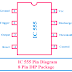## IC 555 Timer

The IC 555 is very useful and popular IC. The IC 555 basically a timer IC. The IC 555 is consists of a timer circuit and the timer circuit contains three five kiloOhm resistors, for this reason, the name of the IC is IC555.

### Pin Diagram of IC 555:

The IC 555 is available in so many packages. Here the block diagram of 8 pin DIP package IC 555 is given below.

#### The function of Each Pin of IC 555:

Pin no.1: The pin 1 provides the ground terminal for the IC to give the power supply.

Pin no.2: The pin 2 is called Trigger. It is connected to the inverting terminal of the second OpAmp or Comparator. As the non-inverting terminal of the second comparator is connected to the 1/3Vcc point so if we decrease the voltage of the trigger pin below the 1/3 Vcc then the output of the comparator will be high and the circuit will be triggered.
Usually, we connect the trigger pin with the Vcc and when we want to change the current state of the comparator we should decrease the voltage of the trigger pin.

Pin no.3: The Pin 3 is called output. The pin 3 is to be connected to the load that means the IC can drive the load through the output pin. The IC can deliver up to 200mA of current through this pin.

Pin no.4: The pin no.4 is used to reset the flip-flop circuit.

Pin no.5: The pin no.5 is directly connected to the inverting terminal of the first comparator. This pin is used to control the input voltage of the first comparator by applying external voltage.

Pin no.6: As the inverting terminal of the first comparator is connected to the 2/3 Vcc point so when the voltage of the non-inverting terminal is greater than2/3 Vcc the output of the first comparator will be high. The pin no.6 or threshold pin is used to give the threshold voltage which is greater than 2/3 Vcc.
Actually, the pin no.2 or Trigger and pin no.6 or Threshold pin are used to control the output of the comparators.

Pin no.7: The pin no.7 is called discharge pin. It is connected to the collector pin of the transistor.

Pin no.8: The pin no.8 or Vcc is used to give power supply to the IC555. The voltage from 4.5V to 15V can be given to the IC555.

### The internal block diagram of IC 555:

You can see in the below figure the internal circuit has no. of blocks. The first block is the voltage divider circuit. The voltage divider circuit is made by three five kiloOhm resistors connected in series. The next block is comparators. Here the comparator using OpAmp is used. There are two comparators in the circuit. The next block is the RS Flip-flop circuit.

The outputs of the comparators are given to the flip-flop circuit. Then the output of the flip-flop circuit is going to the output inverter circuit. The function of the inverter circuit is that it inverse the output of the flip-flop that means if the output of the flip-flop is high then the output of the inverter will be low. The pin no.3 or output pin of the IC is connected to the output of the inverter circuit. A discharge circuit using NPN transistor also connected to the output of the flip-flop circuit.

click on the image to enlarge

### Applications of IC 555:

The IC 555 is mainly used for timing related applications. Like,

1. The IC 555 is used for Tone generation.
2. It is used to make an alarm circuit.
3. They also used for frequency division applications.
4. The IC 555 is used as a relaxation oscillator.
5. They are also used in digital counter circuits.
6. IC 555 is widely used for electronics projects.

IC 555 Applications, Pin Diagram, Internal Circuit Diagram ExplainedReviewed by manoranjan das on 11:07 am Rating: 5